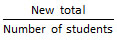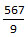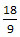# TANCET 2013 Quant Qn 2: Averages

## Question

Medium Quantitative Reasoning

A class had 9 students. In one test, the class average was 61. One student's paper was scored incorrectly, and the resulting score was raised 18 points. What is the corrected class average?

1. 70
2. 63
3. 72
4. 78
5. 74

• ### Step by step solution

1. Number of students in the class = 9
2. Average marks as computed initially = 61
3. Total marks = Average marks * number of students.

Hence, total marks of all 9 students computed with the initial average = 9 * 61 = 549

4. Score for one of the student was raised by 18. This will contribute to the total score of the class increasing by 18 marks.
Therefore, new total marks for the class of 9 students = 549 + 18 = 567

5. The new average === 63

The correct answer is Choice 2.

• ### Alternative Method

The total increases by 18 after the correction is made.

The effect of the increase of 18 in the total will be distributed equally among the 9 numbers when we compute the new average.

So, the effective increase in average will be= 2

Hence, the new average will be 2 more than the initial average. i.e., 2 + 61 = 63.

## Online TANCET MBA CourseTry it Free!

Register in 2 easy steps and start learning in 5 minutes!